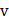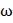# A bullet of mass 0.01 kg and travelling at a speed of 500 m/s strikes a block of 2 kg which is suspended by a string of length 5m. The centre of gravity of the block is found to rise a vertical distance of 0.1m. What is the speed of the bullet after it emerges from the block? a) 200 m/s b) 220 m/s c) 204 m/s d) 284 m/s

## Question ID - 100190 :- A bullet of mass 0.01 kg and travelling at a speed of 500 m/s strikes a block of 2 kg which is suspended by a string of length 5m. The centre of gravity of the block is found to rise a vertical distance of 0.1m. What is the speed of the bullet after it emerges from the block? a) 200 m/s b) 220 m/s c) 204 m/s d) 284 m/s

3537

 (b) Suppose the velocity of the bullet of massisand it strikes the block of mass. After collision, the linear velocity of the block isand that of the bullet isApplying  law of conservation of linear momentum, we getOr  5000.01 = 2+0.01Or  5 = 2+ 0.01(i) Now, the kinetic energy gained by the blockraises it to a heightwhere it gains gravitational potential energyBy conservation of energy, we getOrm/s Putting the value ofin Eq. (i), we getorm/s

Next Question :

A disc of radiusrolls on a horizontal ground with linear accelerationand angular accelerationas shown in Figure. The magnitude of acceleration of pointasshown in the figure at an instant when its linear velocity isand angular velocity iswill bea)b)c)d)